ISEE Middle Level Math : Quadrilaterals

Example Questions

Example Question #11 : Squares

A city block is a perfect square with sides of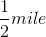.

What is the perimeter of the city block?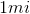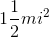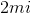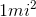Explanation:

A city block is a perfect square with sides of.

What is the perimeter of the city block?

To find the perimeter of a square, simply multiply the side length by 4: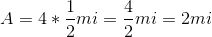So our answer is 2 miles

Example Question #12 : Squares

Find the perimeter of a square with a length of 7in.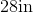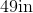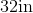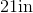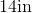Explanation:

To find the perimeter of a square, we will use the following formula: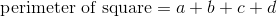where a, b, c, and d are the lengths of the sides of the square.

Now, we know the length of the square is 7in.  Because it is a square, we know all sides are equal.  Therefore, all sides are 7in.

Knowing all of this, we can substitute into the formula.  We get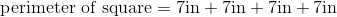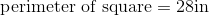Example Question #13 : Squares

Use the following image to answer the question: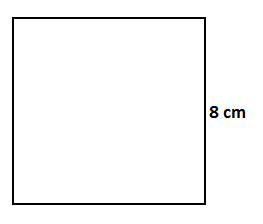Find the perimeter of the square.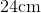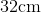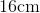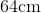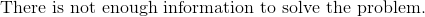Explanation:

To find the perimeter of a square, we will use the following formula:where a, b, c, and d are the lengths of the sides of the square.

Now, let's look at the given square.We can see that one side is 8cm.  Because it is a square, all sides are equal.  Therefore, all sides are 8cm.

Knowing this, we can substitute into the formula.  We get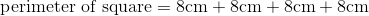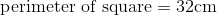Example Question #14 : Squares

Find the perimeter of a square with a length of 9cm.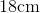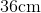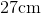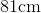Explanation:

To find the perimeter of a square, we will use the following formula:where a, b, c, and d are the lengths of the sides of the square.

Now, we know the length of the square is 9cm.  Because it is a square, all of the sides are equal.  Therefore, all sides are 9cm.

Knowing this, we can substitute into the formula.  We get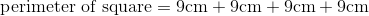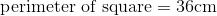Example Question #15 : Squares

Use the following square to answer the question: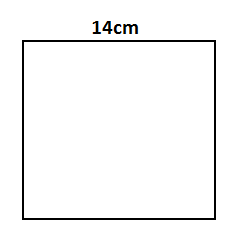Find the perimeter.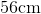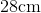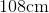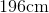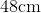Explanation:

To find the perimeter of a square, we will use the following formula:where a, b, c, and d are the lengths of the sides of the square.

Now, given the squareWe can see the width is 14cm.  Because it is a square, all sides are equal.  Therefore, all sides are 14cm.

Knowing this, we can substitute into the formula.  We get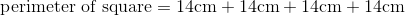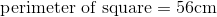Example Question #16 : Squares

Find the perimeter of a square that has a length of 15in.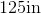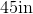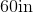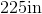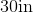Explanation:

To find the perimeter of a square, we will use the following formula:where a, b, c, and d are the lengths of the sides of the square.

Now, we know the length of the square is 15in.  Because it is a square, all sides are equal.  Therefore, all sides are 15 in.

Knowing this, we can substitute into the formula.  We get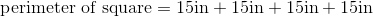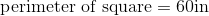Example Question #17 : Squares

Find the perimeter of a square with a length of 24in.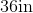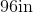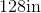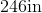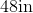Explanation:

To find the perimeter of a square, we will use the following formula:where a, b, c, and d are the lengths of the sides of the square.

Now, we know the length of the square is 24in.  Because it is a square, all sides are equal.  Therefore, all sides are 24in.

Knowing this, we can substitute into the formula.  We get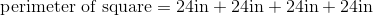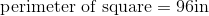Example Question #18 : Squares

Find the perimeter of a square with a width of 19in.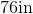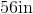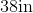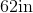Explanation:

To find the perimeter of a square, we will use the following formula: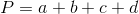where a, b, c, and d are the lengths of the sides of the square.

Now, we know the width of the square is 19in.  Because it is a square, all sides are equal.  Therefore, all sides are 19in.  Knowing this, we can substitute into the formula.  We get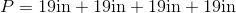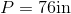Example Question #19 : Squares

Find the perimeter of a square with a width of 8in.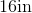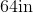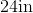Explanation:

To find the perimeter of a square, we will use the following formula:where a, b, c, and d are the lengths of the sides of the square.

Now, we know the width of the square is 8in. Because it is a square, all sides are equal.  Therefore, all sides are 8in.

Knowing this, we can substitute into the formula.  We get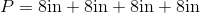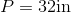Example Question #20 : Squares

The perimeter of a square is 56in.  Find the length of one side.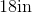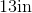Explanation:

To find the perimeter of a square, we use this formula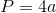where a is the length of one side.  A square has 4 equal sides.  That is why we multiply the length of one side by 4.  To find the length of one side, we will solve for a.

Now, we know the perimeter of the square is 56in.  So, we can substitute.  We get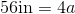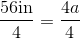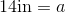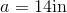Therefore, the length of one side of the square is 14in.